# METHODOLOGIES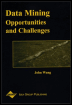Chapter II - Control of Inductive Bias in Supervised Learning Using Evolutionary Computation A Wrapper-Based Approach Data Mining: Opportunities and Challenges by John Wang (ed) Idea Group Publishing 2003Brought to you by Team-Fly

### Metric-Based Model Selection in Time Series Learning

For time series, we are interested in actually identifying a stochastic process from the training data (i.e., a process that generates the observations). The performance element, time series classification, will then apply a model of this process to a continuation of the input (i.e., "test" data) to generate predictions. The question addressed in this section is: "To what degree does the training data (or a restriction of that data to a subset of attributes) probabilistically match a prototype of some known stochastic process?" This is the purpose of metric-based model selection to estimate the degree of match between a subset of the observed data and a known prototype. Prototypes, in this framework, are memory forms (Mozer, 1994), and manifest as embedded patterns generated by the stochastic process that the memory form describes. For example, an exponential trace memory form can express certain types of MA(1) processes. The kernel function for this process is given in Hsu (1998). The more precisely a time series can be described in terms of exponential processes (wherein future values depend on exponential growth or decay of previous values), the more strongly it will match this memory form. The stronger this match, the better the expected performance of an MA(1) learning model, such as an input recurrent (IR) network. Therefore, a metric that measures this degree of match on an arbitrary time series is a useful predictor of IR network performance.

### Control of Representation Bias: A Time-Series Learning Example

Table 1 lists five learning representations, each exemplifying a type of representation or restriction bias for inductive learning from time series, and the metrics corresponding to their strengths. These are referred to as representation metrics because, as documented in the first section (see Figure 1), the choice of representation is local to each node (subnetwork) in the hierarchy, corresponding to a single set within an attribute partition. The choice of hierarchical model is global over the partition, and the corresponding metrics are therefore called representation metrics. Note that this set may be an abstraction, or "merge," of the lowest-level partition used, and is likely to be a refinement, or "split," of the top-level (unpartitioned) set. The metrics are called prescriptive because each one provides evidence in favor of a particular architecture.

Table 1: Five time series representations and their prescriptive metrics

(Time Series) Representation Bias

Representation Metric

Simple recurrent network (SRN)

Exponential trace (AR) score

Time delay neural network (TDNN)

Moving average (MA) score

Gamma network

Autoregressive moving average (ARMA) score

Temporal na ve Bayesian network

Relevance score

Hidden Markov model (HMM)

Test set perplexity

The design rationale is that each metric is based on an attribute chosen to correlate positively (and, to the extent feasible, uniquely) with the characteristic memory form of a time series. A memory form as defined by Mozer (1994) is the representation of some specific temporal pattern, such as a limited-depth buffer, exponential trace, gamma memory (Princip & Lefebvre, 2001), or state transition model.

To model a time series as a stochastic process, one assumes that there is some mechanism that generates a random variable at each point in time. The random variables X(t) can be univariate or multivariate (corresponding to single and multiple attributes or channels of input per exemplar) and can take discrete or continuous values, and time can be either discrete or continuous. For clarity of exposition, the experiments focus on discrete classification problems with discrete time. The classification model is generalized linear regression (Neal, 1996), also known as a 1-of-C coding (Sarle, 2002), or local coding (Kohavi & John, 1997).

Following the parameter estimation literature (Duda et al., 2000), time series learning can be defined as finding the parameters Θ = {θ1, , θn} that describe the stochastic mechanism, typically by maximizing the likelihood that a set of realized or observable values, {x(t1), x(t2), , x(tk)}, were actually generated by that mechanism. This corresponds to the backward, or maximization, step in the expectation-maximization (EM) algorithm (Duda et al., 2000). Forecasting with time series is accomplished by calculating the conditional density P(X(t){Θ, {X(t 1), , X(t m)}}), when the stochastic mechanism and the parameters have been identified by the observable values {x(t)}. The order m of the stochastic mechanism can, in some cases, be infinite; in this case, one can only approximate the conditional density.

Despite recent developments with nonlinear models, some of the most common stochastic models used in time series learning are parametric linear models called autoregressive (AR), moving average (MA), and autoregressive moving average (ARMA) processes.

MA or moving average processes are the most straightforward to understand. First, let {Z(t)} be some fixed zero-mean, unit-variance "white noise" or "purely random" process (i.e., one for which Cov[Z(ti), Z(tj)] = 1 iff ti = tj, 0otherwise). X(t) is an MA(q) process, or "moving average process of order q," if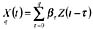, where the βτ are constants. It follows that E[X(t)] = 0 and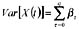. Moving average processes are used to capture "exponential traces" in a time series (Mehrotra et al., 1997; Mozer, 1994; Princip & Lefebvre, 2001). For example, input recurrent neural networks (Ray & Hsu, 1998) are a restricted form of nonlinear MA process model.

AR or autoregressive processes are processes in which the values at time t depend linearly on the values at previous times. With {Z(t)} as defined above, X(t) is an AR(p) process, or "autoregressive process of order p", if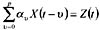, where the αυ are constants. In this case, E[X(t)] = 0, but the calculation of Var[X(t)] depends upon the relationship among the αυ; in general, if ∣αυ 1, then X(t) will quickly diverge. Autoregressive processes are often used to describe stochastic mechanisms that have a finite, short-term, linear "memory"; they are equivalent to infinite-length MA processes constants. Both Jordan recurrent neural networks (Mozer, 1994) and time-delay neural networks (Lang, Waibel, & Hinton., 1990), also known as tapped delay-line neural networks or TDNNs, are a restricted form of nonlinear AR process model (Mehrotra et al., 1997, Princip & Lefebvre, 2001).

ARMA is a straightforward combination of AR and MA processes. With the above definitions, an ARMA(p, q) process is a stochastic process X(t) in which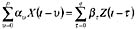, where the {αυ,βτ} are constants (Mozer, 1994). Because it can be shown that AR and MA are of equal expressive power, that is, because they can both represent the same linear stochastic processes, possibly with infinite p or q (Box et al., 1994), ARMA model selection and parameter fitting should be done with specific criteria in mind. For example, it is typically appropriate to balance the roles of the AR(p) and MA(q), and to limit p and q to small constant values (typically 4 or 5) for tractability (Box et al., 1994; Princip & Lefebvre, 2001). The Gamma memory (Princip & deVries, 1992; Princip & Lefebvre, 2001) is a restricted, nonlinear ARMA process model with a neural network architecture and learning rules.

In heterogeneous time series, the embedded temporal patterns belong to different categories of statistical models, such as MA(1) and AR(1). Examples of such embedded processes are presented in the discussion of the experimental test beds. A multichannel time series learning problem can be decomposed into homogeneous subtasks by aggregation or synthesis of attributes. Aggregation occurs in multimodal sensor fusion (e.g., for medical, industrial, and military monitoring), where each group of input attributes represents the bands of information available to a sensor (Stein & Meredith, 1993). In geospatial data mining, these groupings may be topographic. Complex attributes may be synthesized explicitly by constructive induction, as in causal discovery of latent (hidden) variables (Heckerman, 1996); or implicitly by preprocessing transforms (Haykin, 1999; Mehrotra et al., 1997; Ray & Hsu, 1998).

#### Control of Preference Bias: A Data Fusion Example

The learning methods being evaluated define the hierarchical model used to perform multi-strategy learning in the integrated, or composite, learning system. Examples of these are listed in Table 2. Continuing research (Hsu, 1998) also considers the training algorithm to use but is beyond the scope of this chapter. This section presents the metrics for preference bias (the combiner type) and presents hierarchical models for classifier fusion in greater detail.

Table 2: Hierarchical committee machines (combiners) and their prescriptive metrics

Preference Bias (Combiner Type)

Preference Metric

Specialist-Moderator (SM) Network

Factorization score

Multi-strategy Hierarchical Mixture of Experts (MS-HME) Network

Modular mutual information score

The expected performance of a hierarchical model is a holistic measurement; that is, it involves all of the subproblem definitions, the learning architecture used for each one, and even the training algorithm used. It must therefore take into account at least the subproblem definitions. Hence, the metrics used to select a hierarchical model are referred to as preference metrics. Preference metrics in this case are designed to evaluate only the subproblem definitions. This criterion has three benefits: first, it is consistent with the holistic function of hierarchical models; second, it is minimally complex, in that it omits less relevant issues such as the learning architecture for each subproblem from consideration; and third, it measures the quality of an attribute partition. The third property is very useful in heuristic search over attribute partitions; the tree metric can thus serve double duty as an evaluation function for a partition (given a hierarchical model to be used) and for mixture model (given a partitioned data set). As a convention, the choice of partition is committed first; next, the hierarchical model type; then, the learning architectures for each subset, with each selection being made subject to the previous choices.

The preference metric for specialist-moderator networks is the factorization score. The interested reader is referred to Hsu (1998) and Hsu et al. (2000).

#### Multi-strategy Hierarchical Mixture of Experts (MS-HME) Network

The tree metric for HME-type networks is the modular mutual information score. This score measures mutual information across subsets of a partition. It is directly proportional to the conditional mutual information of the desired output given each subset by itself (i.e., the mutual information between one subset and the target class, given all other subsets). It is inversely proportional to the difference between joint and total conditional mutual information (i.e., shared information among all subsets). Let the first quantity be denoted Ii for each subset ai, and the second quantity as I for an entire partition.

The mutual information between discrete random variables X and Y is defined (Cover & Thomas, 1991) as the Kullback-Leibler distance between joint and product distributions: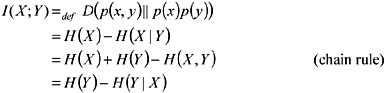The conditional mutual information of X and Y given Z is defined (Cover & Thomas, 1991) as the change in conditional entropy when the value of Z is known: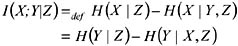The common information of X, Y, and Z (the analogue of k-way intersection in set theory, except that it can have negative value) can now be defined: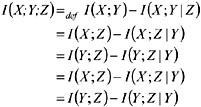The idea behind the modular mutual information score is that it should reward high conditional mutual information between an attribute subset and the desired output given other subsets (i.e., each expert subnetwork will be allotted a large share of the work). It should also penalize high common information (i.e., the gating network is allotted more work relative to the experts). Given these dicta, we can define the modular mutual information for a partition as follows: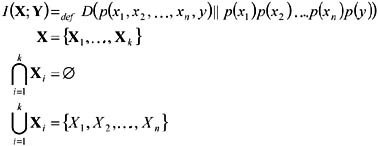which leads to the definition of Ii (modular mutual information) and I (modular common information):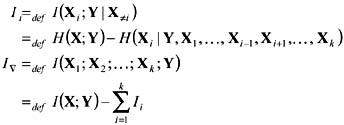Because the desired metric rewards high Ii and penalizes high I, we can define: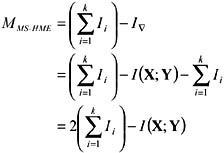### Model Selection and Composite Learning

As explained in the introduction, being able to decompose a learning problem into simpler subproblems still leaves the task of mapping each to its appropriate model the hypothesis language or representation bias (Mitchell, 1997; Witten & Frank, 2000). In the above methodology section, we have just formulated a rationale for using quantitative metrics to accumulate evidence in favor of particular models. This leads to the design presented here, a metric-based selection system for time series learning architectures and general learning methods. Next, we have studied specific time series learning architectures that populate part of a collection of model components, along with the metrics that correspond to each. We then addressed the problem of determining a preference bias (data fusion algorithm) for multi-strategy learning by examining two hierarchical mixture models to see how they can be converted into classifier fusion models that also populate this collection. Finally, we surveyed metrics that correspond to each.

I pause to justify this coarse-grained approach to model selection. As earlier defined, model selection is the problem of choosing a hypothesis class that has the appropriate complexity for the given training data (Hjorth, 1994; Schuurmans, 1997; Stone, 1977). Quantitative or metric-based methods for model selection have previously been used to learn using highly flexible models with many degrees of freedom (Schuurmans, 1997), but with no particular assumptions on the structure of decision surfaces, e.g., that they are linear or quadratic (Geman et al., 1992). Learning without this characterization is known in the statistics literature as model-free estimation or nonparametric statistical inference. A premise of this chapter is that, for learning from heterogeneous time series, indiscriminate use of such models is too unmanageable. This is especially true in diagnostic monitoring applications such as crisis monitoring, because decision surfaces are more sensitive to error when the target concept is a catastrophic event (Hsu et al., 1998).

The purpose of using model selection in decomposable learning problems is to fit a suitable hypothesis language (model) to each subproblem (Engels, Verdenius, & Aha,1998). A subproblem is defined in terms of a subset of the input and an intermediate concept, formed by unsupervised learning from that subset. Selecting a model entails three tasks. The first is finding partitions that are consistent enough to admit at most one "suitable" model per subset. The second is building a collection of models that is flexible enough so that some partition can have at least one model matched to each of its subsets. The third is to derive a principled quantitative system for model evaluation so that exactly one model can be correctly chosen per subset of the acceptable partition or partitions. These tasks indicate that a model selection system at the level of subproblem definition is desirable, because this corresponds to the granularity of problem decomposition, the design choices for the collection of models, and the evaluation function. This is a more comprehensive optimization problem than traditional model selection typically adopts (Geman et al., 1992; Hjorth, 1994), but it is also approached from a less precise perspective, hence the term coarse-grained.

This idea is based upon suggestions by Michael I. Jordan.

 Brought to you by Team-Fly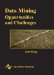Data Mining: Opportunities and Challenges
ISBN: 1591400511
EAN: 2147483647
Year: 2003
Pages: 194
Authors: John Wang